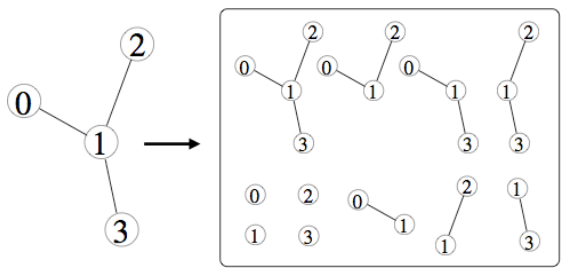시간 제한메모리 제한제출정답맞은 사람정답 비율
2 초 1024 MB15121184.615%

## 문제

Trees are used for all sorts of purposes, such as parsing, information storage and retrieval, sorting, etc. An unweighted, undirected, unrooted tree T is made up of vertices and edges. Each edge connects two vertices. In a tree, any pair of vertices must be connected by some path of edges and vertices through the tree, but they may be connected by only one simple path (no cycles are permitted). Note that any tree with V vertices must have V-1 edges.

There are a lot of trees in computer science, but there are even more than might appear at first glance. This is because every tree is itself made up of one or more subtrees. A subtree S of a tree T is itself a tree, made only from the vertices and edges of T. A subtree must have at least one vertex, and a tree is considered a subtree of itself. Here is an example of a tree with four vertices (the large tree on the left) and its 11 subtrees (the smaller trees in the box on the right):Write a program that, for each given tree, determines the number of subtrees that it has.

## 입력

The input file contains multiple test cases, each describing a tree. Each test case starts with an integer 1 ≤ V ≤ 10 indicating the number of vertices in the tree. Vertices are implicitly labeled 0 through V-1. V is followed by V-1 edge descriptions. Each edge description has two integers 0 ≤ A < V and 0 ≤ B < V, where A ≠ B, indicating the endpoints A and B are connected. The last test case is followed by a line containing a single zero.

## 출력

For each test case, print the case number (beginning with 1) followed by the number of subtrees. Follow the format of the sample output.

## 예제 입력 1

4
0 1
1 2
2 3
4
0 1
1 2
1 3
0


## 예제 출력 1

Case 1: 10
Case 2: 11